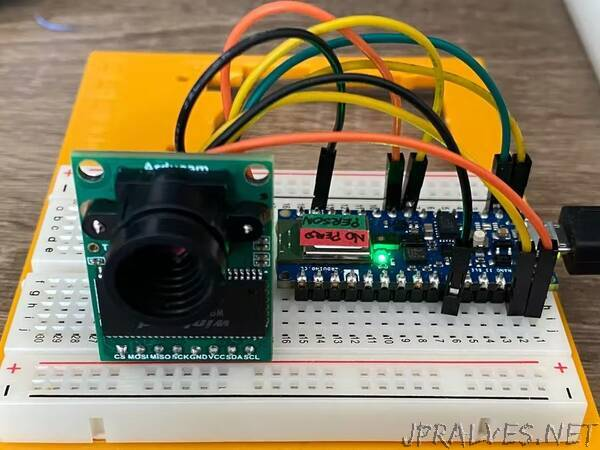Is easy to run large Machine Learning models in the cloud with large computational and memory resources. What about in the Arduino Nano?

Deep Learning Workflow
In this project, the steps followed to implement our person detection application are the book referenced :

Decide the goal –person detection using Arduino Nano BLE 33 and Arducam
Collect a dataset – VisualWake Words (derived from COCO dataset)
Design a ModelArchitecture – Convolutional Neural Networks – MobileNet v1
Train the Model – Training, validation and test
Convert the Model –using TensorFlow Lite Converter
Run Inference – Output data – Person Detected and Not detected scores, green/red light
Evaluate andTroubleshoot – Real world performance
Convert the Model
In a normal environment, TensorFlow is used to build and train large Machine Learning models. A TensorFlow model is a set of instructions that tell an interpreter how to transform data in order to produce an output. To use our model, we load into the memory and execute it using the TensorFlow interpreter.

TensorFlow interpreter is supposed to be run in powerful desktop computers and servers. To run models on microcontrollers, we need a different interpreter for this case.TensorFlow has an interpreter and tools to run models on small-low system powered devices and it’s called “TensorFlow Lite”.

Run Inference
After the model is converted, it can be deployed using the library “TensorFlow Lite forMicrocontrollers C++”. The code takes raw input data from the sensors and transforms it into the same form that the model was trained. This transformed data is passed to the model and run inference.

This result is an output containing predictions. In case of our “person detection” model, the output will be a score for each of our classes “person” and “no person”. These models that classify data, typically the scores for all classes will sum to 1, and the class with the highest score will be the prediction. The higher the difference between the scores, the higher the confidence in the prediction. In our application we are using a score from -100 to +100”